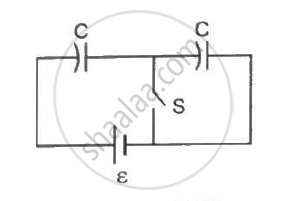Department of Pre-University Education, Karnataka course PUC Karnataka Science Class 12
Share

# Consider the Situation Shown in the Figure. the Switch S Is Open for a Long Time and Then Closed. (A) Find the Charge Flown Through the Battery When the Switch S is Closed. - Physics

ConceptCapacitors and Capacitance

#### Question

Consider the situation shown in the figure. The switch S is open for a long time and then closed. (a) Find the charge flown through the battery when the switch S is closed. (b) Find the work done by the battery.(c) Find the change in energy stored in the capacitors.(d) Find the heat developed in the system.#### Solution

Since the switch is opened for a long time, the capacitor is in series.

therefore C_(eq) = C/2

When the switch is closed, the charge flown from the battery is given by

q = C/2 xx ε =( Cε)/2

(b) Work done, W = q xx V

⇒ q = C_ε/2 xx ε = (Cε^2)/2

(c) The change in the energy stored in the capacitors is given by

ΔE = 1/2 C_(eq) xx V_2

= 1/2 xx C/2 xx ε^2 = 1/4 Cε^2

(d) The heat developed in the system is given by

H = ΔE

⇒ H = 1/4 Cε^2

Is there an error in this question or solution?

#### Video TutorialsVIEW ALL 

Solution Consider the Situation Shown in the Figure. the Switch S Is Open for a Long Time and Then Closed. (A) Find the Charge Flown Through the Battery When the Switch S is Closed. Concept: Capacitors and Capacitance.
S